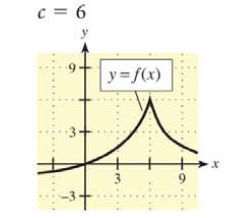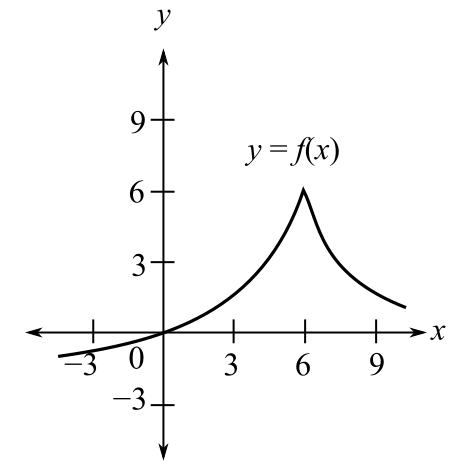Chapter 9.1, Problem 2E### Mathematical Applications for the ...

11th Edition
Ronald J. Harshbarger + 1 other
ISBN: 9781305108042

#### Solutions

Chapter
Section### Mathematical Applications for the ...

11th Edition
Ronald J. Harshbarger + 1 other
ISBN: 9781305108042
Textbook Problem

# In Problems 1 -6, a graph of y   =   f ( x ) is shown and a c-value is given. For each problem, use the graph to find the following, whenever they exist.(a) lim x → c f ( x ) and (b) f ( c ) c = 6(a)

To determine

The value of limxcf(x) by the use of graph,Explanation

Given Information:

The value of c is 6. The graph of y=f(x) is

Explanation:

Consider the provided graph,

The value of c is equal to 6.

The limit from the left is represented by limx6f(x) and the limit from the right is represented by limx6+f(x). The limxcf(x) will exist at c=6 when the limit from the left, that is, the values of f(c) but c<6, is equal to the limit from the right, that is, the values of f(c) but c>6

(b)

To determine

The value of f(c) by the use of the graph,### Still sussing out bartleby?

Check out a sample textbook solution.

See a sample solution

#### The Solution to Your Study Problems

Bartleby provides explanations to thousands of textbook problems written by our experts, many with advanced degrees!

Get Started

#### Evaluate the expression sin Exercises 116. 230

Finite Mathematics and Applied Calculus (MindTap Course List)

#### A sample with a mean of M = 8 has X = 56. How many scores are in the sample?

Essentials of Statistics for The Behavioral Sciences (MindTap Course List)

#### Find y and y. y=eex

Single Variable Calculus: Early Transcendentals

#### True or False: is a convergent series.

Study Guide for Stewart's Single Variable Calculus: Early Transcendentals, 8th